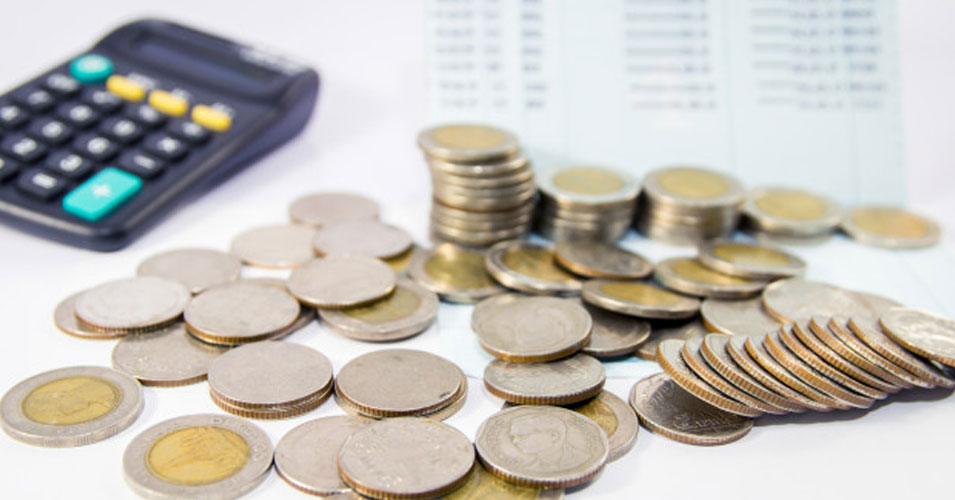• Save

# Compound Interest Questions and Answers for Competitive ExamsIn almost all competitive examinations conducted by the central and state government, are asked questions related to compound interest under the subject of Mathematics. In the questions based on compound interest, interest is calculated on interest based on principal. Students have to need special knowledge of principal and simple interest to solve questions related to compound interest, as these questions cannot be solved without understanding the formulas.

Here today, I have provided questions on compounding interest to the students who are preparing for competitive examinations. Also with the help of this article, you can continuously practice the questions given for better results in the exam.

You can also Practice with: Compound Interest Formula with Examples.

## Important Compound Interest Questions

Q :

A man borrowed some money from a private organisation at 5 % simple interest per annum. He landed this money to another person at 10% compound interest per annum, and made a profit of Rs. 26,410 in 4 years. The man borrowed

(A) 132050

(B) 100000

(C) 200000

(D) 150000

Q :

A sum of Rs. 2000 amounts to Rs. 4000 in two years at compound interest. In how many years does the same amount becomes Rs. 8000.

(A) 6

(B) 8

(C) 2

(D) 4

Q :

A sum of money becomes eight times of itself in 3 years at compound interest. The rate of interest per annum is

(A) 20 %

(B) 10 %

(C) 100 %

(D) 80 %

Q :

A sum of money is invested at 20 % compound interest (compounded annually). It would fetch 723 more if interest is compound half-yearly. The sum is –

(A) ₹ 20,000

(B) ₹ 7,500

(C) ₹ 72,300

(D) ₹ 30,000

Q :

The amount on ₹ 25000 in 2 years at annually compound interest. if the rate for the successive years be 4 % and 5 % per annum respectively is

(A) ₹ 28500

(B) ₹ 30000

(C) ₹ 26800

(D) ₹ 27300

Q :

A sum of money becomes eight times in 3 years, if the rate is compounded annually. In how much time will the amount at the same compound rate become sixteen times?

(A) 8 years

(B) 5 years

(C) 6 years

(D) 4 years

Q :

In 3 years ₹ 3000 amounts to ₹ 3993 at x % compound interest, compounded annually. The value of x is

(A) 5%

(B) 33%

(C) 10%

(D) 8%

Q :

In what time will ₹ 64,000 amount to ₹ 68,921 at 5 % per annum interest being compounded half yearly?

(A) $$3{1\over 2}\ years$$

(B) $$2{1\over 2}\ years$$

(C) $$1{1\over 2}\ years$$

(D) $$1{3\over 2}\ years$$

Q :

A sum of Rs. 8000 will amount to Rs. 8820 in 2 years if the interest is calculated every year. The rate of compound interest is

(A) 3 %

(B) 5 %

(C) 6 %

(D) 7 %

Q :

The compound interest earned in two years at 12% per annum Rs 10176. What is the sum (in Rs) invested?

(A) 40000

(B) 80000

(C) 50000

(D) 60000